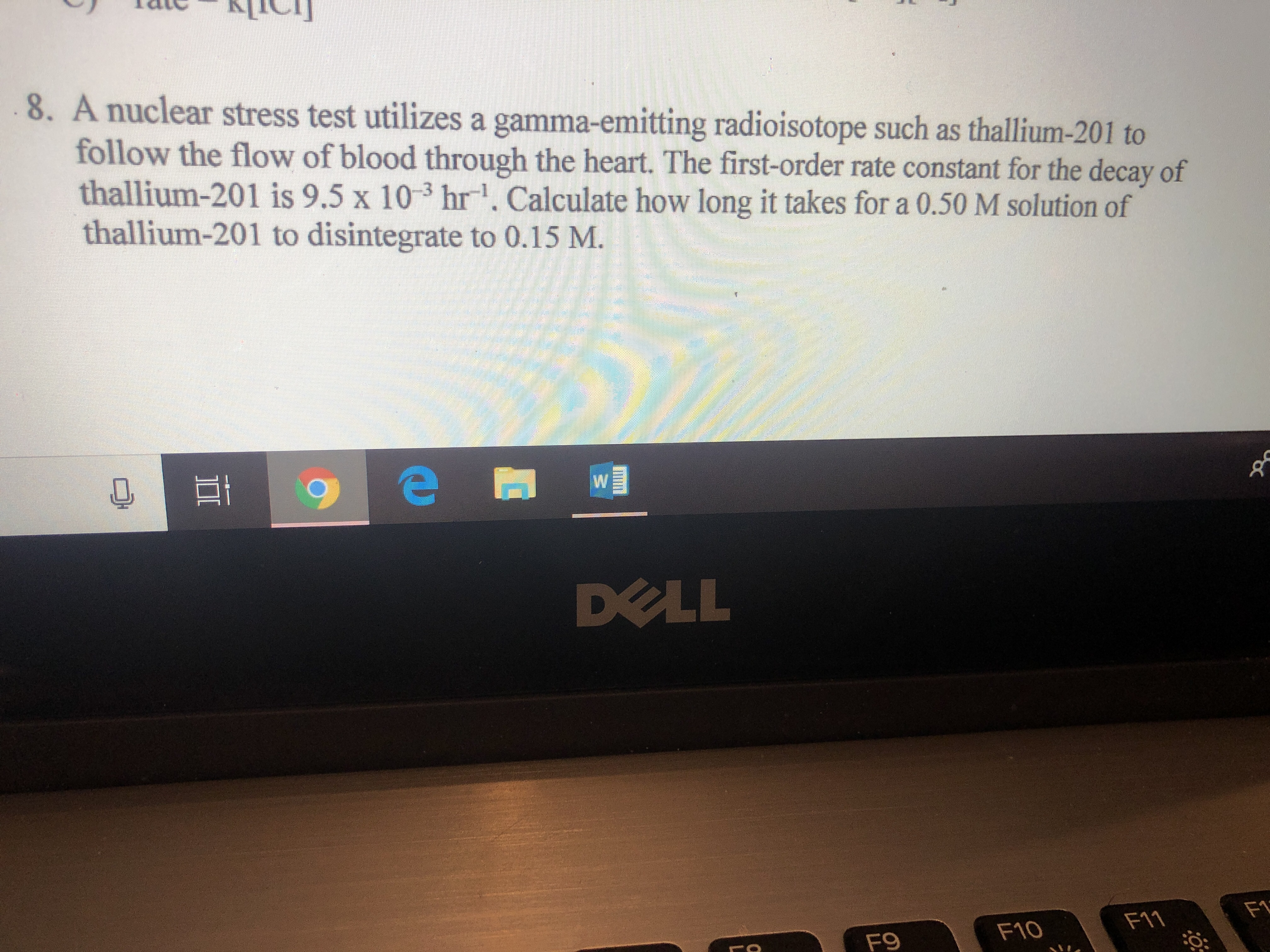# 8. A nuclear stress test utilizes a gamma-emitting radioisotope such as thallium-201 to follow the flow of blood through the heart. Th first-order rate constant for the decay of thallium-201 is 9.5 x 10 3 hr . Calculate how long it takes for a 0.50 M solution of thallium-201 to disintegrate to 0.15 M. DOLL F11 F9 F10

Questionhelp_outlineImage Transcriptionclose8. A nuclear stress test utilizes a gamma-emitting radioisotope such as thallium-201 to follow the flow of blood through the heart. Th first-order rate constant for the decay of thallium-201 is 9.5 x 10 3 hr . Calculate how long it takes for a 0.50 M solution of thallium-201 to disintegrate to 0.15 M. DOLL F11 F9 F10 fullscreen

### Want to see this answer and more?

Experts are waiting 24/7 to provide step-by-step solutions in as fast as 30 minutes!*